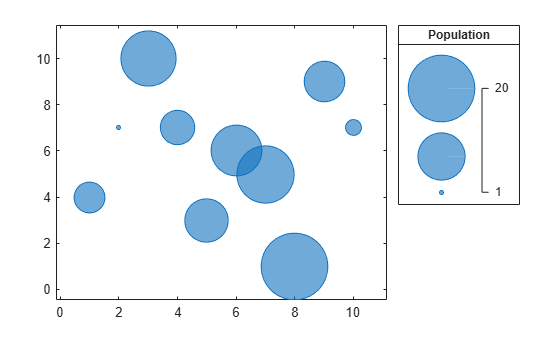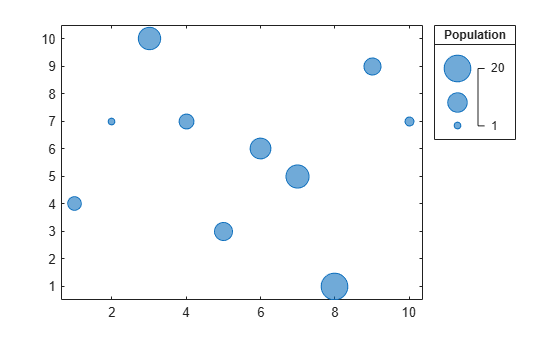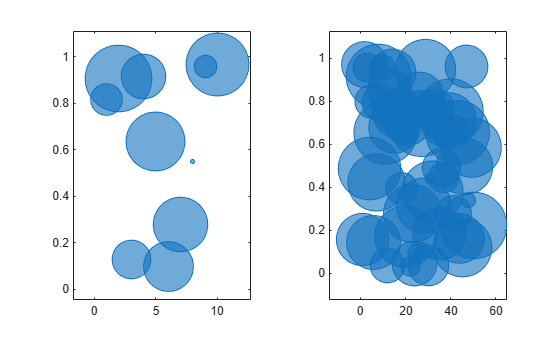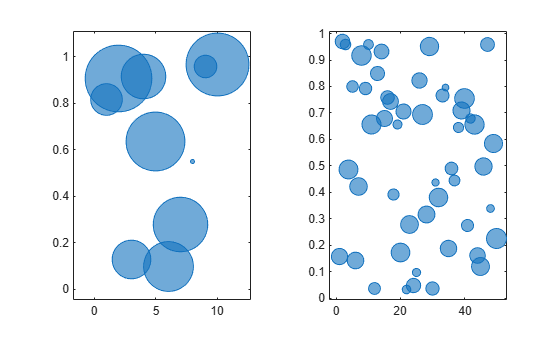# bubblesize

Set minimum and maximum bubble sizes in points

## Syntax

``bubblesize(sizerange)``
``bubblesize(ax,sizerange)``
``sz = bubblesize``
``sz = bubblesize(ax)``

## Description

example

````bubblesize(sizerange)` sets the size of the smallest and largest bubble for bubble charts in the current axes. Specify `sizerange` as a two-element vector containing the smallest and largest diameters in points, where 1 point = 1/72 inch.```

example

````bubblesize(ax,sizerange)` sets the bubble sizes in the axes specified by `ax`.```

example

````sz = bubblesize` returns the size range for the current axes as a two-element vector containing the diameters of the smallest and largest bubbles in points.```

example

````sz = bubblesize(ax)` returns the size range for the axes specified by `ax`.```

## Examples

collapse all

Create a bubble chart with a legend.

```x = 1:10; y = [4 7 10 7 3 6 5 1 9 7]; sz = [5 1 14 6 9 12 15 20 8 2]; bubblechart(x,y,sz); bubblelegend('Population','Location','northeastoutside')```Get the current range of bubble sizes.

`bubblesize`
```ans = 1×2 3 50 ```

Change the bubble sizes so that they are between `5` and `20` points in diameter. The bubble legend automatically updates to reflect the new bubble sizes.

`bubblesize([5 20])`Create two sets of data, then create a tiled chart layout containing two bubble charts.

```% Create two sets of data x1 = 1:10; x2 = 1:50; y1 = rand(1,10); y2 = rand(1,50); sz1 = randperm(10,10); sz2 = randperm(50,50); % Create tiled chart layout t = tiledlayout(1,2); % Create bubble chart in first tile ax1 = nexttile; bubblechart(x1,y1,sz1) % Create bubble chart in first tile ax2 = nexttile; bubblechart(x2,y2,sz2)```Query the range of bubble sizes in the right chart by passing `ax2` to the `bubblesize` function.

`bubblesize(ax2)`
```ans = 1×2 3 50 ```

Change the bubble sizes in the right chart so that they range between `5` and `15` points in diameter.

`bubblesize(ax2,[5 15])`## Input Arguments

collapse all

Size range, specified as a two-element vector containing positive numbers, where the second number is greater than the first. The numbers specify the smallest and largest bubble sizes in points, where 1 point = 1/72 inch.

By default, bubble charts use a size range of `[3 50]`.

Example: `bubblesize([20 70])` sets the smallest bubble size to 20 points and the largest bubble size to 70 points.

Target axes, specified as an `Axes`, `PolarAxes`, or `GeographicAxes` object.